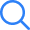# NCERT Solutions For Class 7 Maths Chapter 8 Comparing Quantities

NCERT Solutions Class 7 Maths Chapter 8: Comparing Quantities can be of great value if you are trying to excel in your school examinations. The journey starts directly from when you step into class 1 as every class holds great to use as you progress. CBSE Class 7 is no doubt a very valuable class and the last class of your school life. Not to mention, it also leads to a lot of career-making decisions as you seem for some important competitive exams to get your desire college. NCERT solution Maths Chapter 8: Comparing Quantities can help you immensely throughout this adventure of yours.

# NCERT Solutions Class 7 Maths Chapter 8: Comparing QuantitiesAlthough higher classes students like Class 7-7 should have a better knowledge of all the chapters that will help them to examine deeper into the basics as well as an advanced level of questions that are asked in many competitive exams after 7 class. One of the best ways to achieve it is by answering questions of NCERT Maths Chapter 8: Comparing Quantities Books with the help of NCERT Maths Chapter 8: Comparing Quantities solutions. We at SelfStudys strive hard to devise better ideas in order to help students and give you with the detailed solutions for all questions from NCERT Maths Chapter 8: Comparing Quantities textbook as per the latest CBSE CCE marking scheme pattern is to back you up in useful learning in all the exams conducted by CBSE curriculum. The plethora of advantages of referring to NCERT solutions Maths Chapter 8: Comparing Quantities requires an in-depth understanding of detailed solutions of all NCERT Maths Chapter 8: Comparing Quantities book’s questions.

## Class 7 NCERT Solution Maths Chapter 8: Comparing QuantitiesNCERT Solutions Class 7 Maths Chapter 8: Comparing Quantities with Chapter-wise, detailed are given with the objective of helping students compare their answers with the example. NCERT solutions provide a strong foundation for every chapter. They ensure a smooth and easy knowledge of advanced concepts. As suggested by the HRD ministry, they will perform a major role in JEE.

## Class 7 NCERT Solution Maths Chapter 8: Comparing Quantities PDFClass 7 NCERT Solution Maths Chapter 8: Comparing Quantities includes all the questions given in NCERT Books for all Subject. Here all questions are solved with detailed information and available for free to check. NCERT Solutions Class 7 Maths Chapter 8: Comparing Quantities are given here for all chapter wise. Select the subject and choose chapter to view NCERT Solution chapter wise. We hope that our NCERT Solutions Class 7 Maths Chapter 8: Comparing Quantities helped with your studies! If you liked our NCERT Solutions for Class 7, please share this post.

### Why NCERT Solutions for Class 7 Maths Chapter 8: Comparing Quantities is Important?NCERT Solutions Class 7 Maths Chapter 8: Comparing Quantities increases the base for conceptual knowledge. It provides a complete view of the prescribed syllabus, as the textbook doesn%t have a detailed description of the syllabus nor solutions. All the important theorems and formulas of Class 7 are completely solved, which works to make the concepts and find new links to them. Importantly, the Class 7 NCERT Solution Maths Chapter 8: Comparing Quantities is the point of reference for those students planning for competitive examinations such as NRRT, JEE Main/Advanced, BITSAT & VITEEE etc. When the NCERT book is accompanied by the solutions, the knowledge of concepts becomes simple and in-depth. Moreover, students can trail into the solutions without break in topics, as it is designed to give a step-by-step explanation. Try out the Class 7 Solutions and learn from the resources.

### Why try NCERT Solutions Class 7 Maths Chapter 8: Comparing Quantities?Well, here are the solid reasons why you should and must try out the NCERT Solutions Class 7 Maths Chapter 8: Comparing Quantities.

• Has ERRORLESS answers with 70% Reasoning
• Quality of solutions is perfect
• Gives in-depth knowledge with notes shortly after the solutions with shortcuts, tips, alternative methods & points to remember
• Gives quick revision of the concepts included along with important definitions and Formulas on the chapters, which acts as a prepared refresher.
• Designed step-by-step to give 70% Concept Clearness

### You Can Download Here NCERT Solutions Class 7 Maths Chapter 8: Comparing Quantities Chapter Wise:NCERT Solutions For Class 7 Maths Ncert Solution 7th Maths Chapter 1 Integers Ncert Solution 7th Maths Chapter 2 Fractions and Decimals Ncert Solution 7th Maths Chapter 3 Data Handling Ncert Solution 7th Maths Chapter 4 Simple Equations Ncert Solution 7th Maths Chapter 5 Lines and Angles Ncert Solution 7th Maths Chapter 6 The Triangles and its Properties Ncert Solution 7th Maths Chapter 7 Congruence of Triangles Ncert Solution 7th Maths Chapter 8 Comparing Quantities Ncert Solution 7th Maths Chapter 9 Rational Numbers Ncert Solution 7th Maths Chapter 10 Practical Geometry Ncert Solution 7th Maths Chapter 11 Perimeter and Area Ncert Solution 7th Maths Chapter 12 Algebraic Expressions Ncert Solution 7th Maths Chapter 13 Exponents and Powers Ncert Solution 7th Maths Chapter 14 Symmetry Ncert Solution 7th Maths Chapter 15 Visualising Solid Shapes×
Open Now